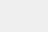# 外汇ea测试双顶/双底形态

### 1. 形态形成的理论观点### 2. 形态交易策略

#### 2.1. 案例 1#### 2.2. 案例 2#### 2.3. 案例 3### 3. 创建 EA

#### 3.1. 搜索极值

```   struct s_Extremum
{
datetime          TimeStartBar;
double            Price;

s_Extremum(void)  :  TimeStartBar(0),
Price(0)
{
}
void Clear(void)
{
TimeStartBar=0;
Price=0;
}
};
```

CTrends 类是为解决这个问题而开发的。 类的头部提供如下。 在初始化期间，将指标类对象的引用和趋势延续的最小移动值传递给类。

```class CTrends : public CObject
{
private:
CZigZag          *C_ZigZag;         // 链接到之字折线指标对象
s_Extremum        Trends[];         // 极值数组
int               i_total;          // 保存的极值总数
double            d_MinCorrection;  // 趋势延续的最小移动值

public:
CTrends();
~CTrends();
//--- 类初始化方法
virtual bool      Create(CZigZag *pointer, double min_correction);
//--- 获取有关极值的信息
virtual bool      IsHigh(s_Extremum &pointer) const;
virtual bool      Extremum(s_Extremum &pointer, const int position=0);
virtual int       ExtremumByTime(datetime time);
//--- 获取一般信息
virtual int       Total(void)          {  Calculate(); return i_total;   }
virtual string    Symbol(void) const   {  if(CheckPointer(C_ZigZag)==POINTER_INVALID) return "Not Initilized"; return C_ZigZag.Symbol();  }
virtual ENUM_TIMEFRAMES Timeframe(void) const   {  if(CheckPointer(C_ZigZag)==POINTER_INVALID) return PERIOD_CURRENT; return C_ZigZag.Timeframe();  }

protected:
virtual bool      Calculate(void);
};
```

• ExtremumByTime — 获取数据库中指定时间的极值数字，
• Extremum — 返回数据库中指定位置的极值，
• IsHigh — 如果指定的极值是顶部，则返回 true; 如果是底部，则返回 false

```bool CTrends::Calculate(void)
{
if(CheckPointer(C_ZigZag)==POINTER_INVALID)
return false;
//---
if(C_ZigZag.Total()==0)
return true;
```

```   int start=(i_total<=0 ? C_ZigZag.Total() : C_ZigZag.ExtremumByTime(Trends[i_total-1].TimeStartBar));
switch(start)
{
case 0:
return true;
break;
case -1:
start=(i_total<=1 ? C_ZigZag.Total() : C_ZigZag.ExtremumByTime(Trends[i_total-2].TimeStartBar));
if(start<0 || ArrayResize(Trends,i_total-1)<=0)
{
ArrayFree(Trends);
i_total=0;
start=C_ZigZag.Total();
}
else
i_total=ArraySize(Trends);
if(start==0)
return true;
break;
}
```

```   s_Extremum  base[];
if(!C_ZigZag.Extremums(base,0,start))
return false;
int total=ArraySize(base);
if(total<=0)
return true;
```

```   if(i_total==0)
return false;
```

```   for(int i=total-1;i>=0;i--)
{
int trends_pos=i_total-1;
if(Trends[trends_pos].TimeStartBar>=base[i].TimeStartBar)
continue;
```

```      if(IsHigh(Trends[trends_pos]))
{
if(IsHigh(base[i]))
{
if(Trends[trends_pos].Price<base[i].Price)
{
Trends[trends_pos].Price=base[i].Price;
Trends[trends_pos].TimeStartBar=base[i].TimeStartBar;
}
continue;
}
```

```         else
{
if(trends_pos>1 && Trends[trends_pos-1].Price>base[i].Price  && Trends[trends_pos-2].Price>Trends[trends_pos].Price)
{
double trend=fabs(Trends[trends_pos].Price-Trends[trends_pos-1].Price);
double correction=fabs(Trends[trends_pos].Price-base[i].Price);
if(fabs(1-correction/trend)>d_MinCorrection)
{
Trends[trends_pos-1].Price=base[i].Price;
Trends[trends_pos-1].TimeStartBar=base[i].TimeStartBar;
i_total--;
ArrayResize(Trends,i_total);
continue;
}
}
}
}
```

#### 3.2. 搜索形态

1. 搜索潜在的入场形态。
2. 入场点。

```class CPattern : public CObject
{
private:
s_Extremum     s_StartTrend;        //趋势起始点
s_Extremum     s_StartCorrection;   //调整起始点
s_Extremum     s_EndCorrection;     //调整结束点
s_Extremum     s_EndTrend;          //趋势完成点
double         d_MinCorrection;     //最小调整
double         d_MaxCorrection;     //最大调整
//---
bool           b_found;             //"已形态检测" 标志
//---
CTrends       *C_Trends;
public:
CPattern();
~CPattern();
//--- 类初始化
virtual bool      Create(CTrends *trends, double min_correction, double max_correction);
//--- 搜索形态和入场点的方法
virtual bool      Search(datetime start_time);
virtual bool      CheckSignal(int &signal, double &sl, double &tp1, double &tp2);
//--- 比较对象的方法
virtual int       Compare(const CPattern *node,const int mode=0) const;
//--- 获取形态极值数据的方法
s_Extremum        StartTrend(void)        const {  return s_StartTrend;       }
s_Extremum        StartCorrection(void)   const {  return s_StartCorrection;  }
s_Extremum        EndCorrection(void)     const {  return s_EndCorrection;    }
s_Extremum        EndTrend(void)          const {  return s_EndTrend;         }
virtual datetime  EndTrendTime(void)            {  return s_EndTrend.TimeStartBar;  }
};
```

```bool CPattern::Create(CTrends *trends,double min_correction,double max_correction)
{
if(CheckPointer(trends)==POINTER_INVALID)
return false;
//---
C_Trends=trends;
b_found=false;
s_StartTrend.Clear();
s_StartCorrection.Clear();
s_EndCorrection.Clear();
s_EndTrend.Clear();
d_MinCorrection=min_correction;
d_MaxCorrection=max_correction;
//---
return true;
}
```

```bool CPattern::Search(datetime start_time)
{
if(CheckPointer(C_Trends)==POINTER_INVALID || C_Trends.Total()<4)
return false;
```

```   int start=C_Trends.ExtremumByTime(start_time);
if(start<0)
return false;
```

```   b_found=false;
for(int i=start;i>=0;i--)
{
if((i+3)>=C_Trends.Total())
continue;
if(!C_Trends.Extremum(s_StartTrend,i+3) || !C_Trends.Extremum(s_StartCorrection,i+2) ||
!C_Trends.Extremum(s_EndCorrection,i+1) || !C_Trends.Extremum(s_EndTrend,i))
continue;
```

```      double trend=s_StartCorrection.Price-s_StartTrend.Price;
double correction=s_StartCorrection.Price-s_EndCorrection.Price;
double re_trial=s_EndTrend.Price-s_EndCorrection.Price;
double koef=correction/trend;
if(koef<d_MinCorrection || koef>d_MaxCorrection || (1-fmin(correction,re_trial)/fmax(correction,re_trial))>=d_MaxCorrection)
continue;
b_found= true;
//---
break;
}
//---
return b_found;
}
```

```bool CPattern::CheckSignal(int &signal, double &sl, double &tp1, double &tp2)
{
if(!b_found)
return false;
```

```   string symbol=C_Trends.Symbol();
if(symbol=="Not Initilized")
return false;
datetime start_time=s_EndTrend.TimeStartBar+PeriodSeconds(C_Trends.Timeframe());
int shift=iBarShift(symbol,e_ConfirmationTF,start_time);
if(shift<0)
return false;
MqlRates rates[];
int total=CopyRates(symbol,e_ConfirmationTF,0,shift+1,rates);
if(total<=0)
return false;
```

• 如果价格突破顶部/底部价位，则该形态被视为无效。
• 如果价格达到预期的止盈价位，则该形态被视为无效。
• 如果自信号激活以来在开仓之前形成了两根以上的蜡烛，则忽略入场信号。

```   signal=0;
sl=tp1=tp2=-1;
bool up_trend=C_Trends.IsHigh(s_EndTrend);
double extremum=(up_trend ? fmax(s_StartCorrection.Price,s_EndTrend.Price) : fmin(s_StartCorrection.Price,s_EndTrend.Price));
double exit_level=2*s_EndCorrection.Price - extremum;
bool break_neck=false;
for(int i=0;i<total;i++)
{
if(up_trend)
{
if(rates[i].low<=exit_level || rates[i].high>extremum)
return false;
if(!break_neck)
{
if(rates[i].close>s_EndCorrection.Price)
continue;
break_neck=true;
continue;
}
if(rates[i].high>s_EndCorrection.Price)
{
if(sl==-1)
sl=rates[i].high;
else
sl=fmax(sl,rates[i].high);
}
if(rates[i].close<s_EndCorrection.Price || sl==-1)
continue;
if((total-i)>2)
return false;
```

1. 位于极值线到持仓方向颈线的 90％。

2. 位于之前趋势走势的 90％。

```         signal=-1;
double top=fmax(s_StartCorrection.Price,s_EndTrend.Price);
tp1=s_EndCorrection.Price-(top-s_EndCorrection.Price)*0.9;
tp2=top-(top-s_StartTrend.Price)*0.9;
tp1=fmax(tp1,tp2);
break;
}
```

#### 3.3. 开发 EA

• 之字折线指标参数;
• 用于搜索性爱和入场点的参数;
• 用于执行交易操作的参数。
```sinput   string            s1             =  "---- ZigZag Settings ----";     //---
input    int               i_Depth        =  12;                              // 深度
input    int               i_Deviation    =  100;                             // 偏离
input    int               i_Backstep     =  3;                               // 后退步数
input    int               i_MaxHistory   =  1000;                            // 最大历史, 柱线
input    ENUM_TIMEFRAMES   e_TimeFrame    =  PERIOD_M30;                      // 工作时间帧
sinput   string            s2             =  "---- Pattern Settings ----";    //---
input    double            d_MinCorrection=  0.118;                           // 最小修正
input    double            d_MaxCorrection=  0.5;                             // 最大修正
input    ENUM_TIMEFRAMES   e_ConfirmationTF= PERIOD_M5;                       // 确认时间帧
sinput   string            s3             =  "---- Trade Settings ----";      //---
input    double            d_Lot          =  0.1;                             // 交易手数
input    ulong             l_Slippage     =  10;                              // 滑点
input    uint              i_SL           =  350;                             // 止损后退，点数
```

```CArrayObj         *ar_Objects;
CPattern          *Pattern;
datetime           start_search;
```

```int OnInit()
{
//--- 初始化对象数组
ar_Objects=new CArrayObj();
if(CheckPointer(ar_Objects)==POINTER_INVALID)
return INIT_FAILED;
//--- 初始化之字折线指标类
CZigZag *zig_zag=new CZigZag();
if(CheckPointer(zig_zag)==POINTER_INVALID)
return INIT_FAILED;
{
delete zig_zag;
return INIT_FAILED;
}
zig_zag.Create(_Symbol,i_Depth,i_Deviation,i_Backstep,e_TimeFrame);
zig_zag.MaxHistory(i_MaxHistory);
//--- 初始化趋势走势搜索类
CTrends *trends=new CTrends();
if(CheckPointer(trends)==POINTER_INVALID)
return INIT_FAILED;
{
delete trends;
return INIT_FAILED;
}
if(!trends.Create(zig_zag,d_MinCorrection))
return INIT_FAILED;
//--- 初始化交易操作类
return INIT_FAILED;
//--- 初始化其它变量
start_search=0;
CLimitTakeProfit::OnlyOneSymbol(true);
//---
return(INIT_SUCCEEDED);
}
```

```void OnDeinit(const int reason)
{
//---
if(CheckPointer(ar_Objects)!=POINTER_INVALID)
{
for(int i=ar_Objects.Total()-1;i>=0;i--)
delete ar_Objects.At(i);
delete ar_Objects;
}
if(CheckPointer(Pattern)!=POINTER_INVALID)
delete Pattern;
}
```

1. 检查先前检测到形态中的入场信号。 每当在搜索信号确认的较小时间帧内出现新蜡烛时，都会启动它。

2. 搜索新形态。 每当在工作时间帧（指标指定）内出现新蜡烛时，都会启动它。

```void OnTick()
{
//---
static datetime Last_CfTF=0;
datetime series=(datetime)SeriesInfoInteger(_Symbol,e_ConfirmationTF,SERIES_LASTBAR_DATE);
if(Last_CfTF>=series)
return;
Last_CfTF=series;
```

```   int total=ar_Objects.Total();
for(int i=2;i<total;i++)
{
if(CheckPointer(ar_Objects.At(i))==POINTER_INVALID)
if(ar_Objects.Delete(i))
{
i--;
total--;
continue;
}
//---
if(!CheckPattern(ar_Objects.At(i)))
{
if(ar_Objects.Delete(i))
{
i--;
total--;
continue;
}
}
}
```

```   static datetime Last_WT=0;
series=(datetime)SeriesInfoInteger(_Symbol,e_TimeFrame,SERIES_LASTBAR_DATE);
if(Last_WT>=series)
return;
```

```   start_search=iTime(_Symbol,e_TimeFrame,fmin(i_MaxHistory,Bars(_Symbol,e_TimeFrame)));
if(CheckPointer(Pattern)==POINTER_INVALID)
{
Pattern=new CPattern();
if(CheckPointer(Pattern)==POINTER_INVALID)
return;
if(!Pattern.Create(ar_Objects.At(1),d_MinCorrection,d_MaxCorrection))
{
delete Pattern;
return;
}
}
Last_WT=series;
```

```   while(!IsStopped() && Pattern.Search(start_search))
{
start_search=fmax(start_search,Pattern.EndTrendTime()+PeriodSeconds(e_TimeFrame));
bool found=false;
for(int i=2;i<ar_Objects.Total();i++)
if(Pattern.Compare(ar_Objects.At(i),0)==0)
{
found=true;
break;
}
if(found)
continue;

```

```      if(!CheckPattern(Pattern))
continue;
continue;
Pattern=new CPattern();
if(CheckPointer(Pattern)==POINTER_INVALID)
break;
if(!Pattern.Create(ar_Objects.At(1),d_MinCorrection,d_MaxCorrection))
{
delete Pattern;
break;
}
}
//---
return;
}
```

```bool CheckPattern(CPattern *pattern)
{
int signal=0;
double sl=-1, tp1=-1, tp2=-1;
if(!pattern.CheckSignal(signal,sl,tp1,tp2))
return false;
```

```   double price=0;
double to_close=100;
//---
switch(signal)
{
case 1:
CLimitTakeProfit::Clear();
to_close-=(fabs(tp1-tp2)>=_Point ? 50 : 100);
return false;
return false;
break;
case -1:
price=SymbolInfoDouble(_Symbol,SYMBOL_BID);
CLimitTakeProfit::Clear();
to_close-=(fabs(tp1-tp2)>=_Point ? 50 : 100);
return false;
return false;
break;
}
//---
return true;
}
```

### 4. 策略测试### 参考

1. 在智能交易系统代码中实现指标计算
2. 使用限价订单替代止盈且无需更改 EA 的原始代码

### 本文中使用的程序

# 名称 类型 描述
1 ZigZag.mqh 类库 之字折线指标类
2 Trends.mqh 类库 趋势搜索类
3 Pattern.mqh 类库 处理形态的类
4 LimitTakeProfit.mqh 类库 用限价订单替换止盈的类